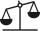Coal storage

The coal storage distribute received coal shipment within three days. The first day distribute third of the shipments, the second day of two-fifths of the rest and the third day 300 tons of coal. How many tons of coal distributed first and second day?

Result

a =  250 t
b =  200 t

Solution:

a+b+300 = t
a = t/3
b = 2/5(t - a)

a+b-t = -300
3a-t = 0
2a+5b-2t = 0

a = 250
b = 200
t = 750

Calculated by our linear equations calculator.

Leave us a comment of example and its solution (i.e. if it is still somewhat unclear...):Be the first to comment!To solve this verbal math problem are needed these knowledge from mathematics:

Need help calculate sum, simplify or multiply fractions? Try our fraction calculator. Do you have a linear equation or system of equations and looking for its solution? Or do you have quadratic equation?

Next similar examples:

1. Dropped sheetsThree consecutive sheets dropped from the book. The sum of the numbers on the pages of the dropped sheets is 273. What number has the last page of the dropped sheets?
2. ClassroomOne-eighth of 9th class was interested in studying at a grammar school, at a business academy one sixth, at secondary vocational schools quarter, to SOU one third and the remaining three students were interested in the school of art direction. How many st
3. Unknown number 11That number increased by three equals three times itself?
4. Equation 15Solve equation with variables on both sides:
5. EqnSolve equation with fractions: 2x/3-50=40+x/4
6. FruitsAmy bought a basket of fruits 1/5 of them were apples,1/4 were oranges, and the rest were 33 bananas. How many fruits did she buy in all?
7. Equation 29Solve next equation: 2 ( 2x + 3 ) = 8 ( 1 - x) -5 ( x -2 )
8. Unknown number 6Determine x if 1/6 of x is equal to 2/5 of the number 24.
9. Simple equationSolve the following simple equation: 2. (4x + 3) = 2-5. (1-x)
10. Write 2Write 791 thousandths as fraction in expanded form.
11. TeacherTeacher Rem bought 360 pieces of cupcakes for the outreach program of their school. 5/9 of the cupcakes were chocolate flavor and 1/4 wete pandan flavor and the rest were vanilla flavor. How much more chocolate flavor cupcakes than vanilla flavor?
12. EquationSolve equation and check the result: 1.4x - 3/2 + x - 9,8 = x + 0,4/3 - 7 + 1,6/6
13. ZdeněkZdeněk picked up 15 l of water from a 100-liter full-water barrel. Write a fraction of what part of Zdeněk's water he picked.
14. Passenger boatTwo-fifths of the passengers in the passenger boat were boys. 1/3 of them were girls and the rest were adult. If there were 60 passengers in the boat, how many more boys than adult were there?
15. BrickIsosceles scale has on one side all brick and second weight 1 kg and 1/4 of brick. The balance is in equilibrium. What is the weight of a brick?
16. The bunsKate, Zofia and Peter Liked buns. Even today, their grandmother prepare their favorite meal. Katka eats 4 bunches, Žofia 3 and Petra eats 5 buns. Their grandmother said to them, "My inmate will you know how many buns I have been make today, if those you ea
17. Trees3/5 trees are apples, cherries are 1/3. 5 trees are pear. How many is the total number of trees?